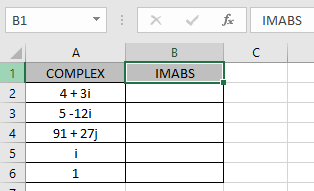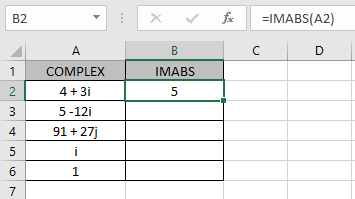# How to use the IMABS Function in Excel

COMPLEX number (inumber) in excel derived for mathematical number having real and imaginary coefficients. In mathematics we call it the coefficient of i or j.
i = √-1
Square root of negative number is not possible, so for calculation purpose, √-1 is named as imaginary and call it iota (i or j). For calculation of some term like shown below.

= 2 +√-25

= 2 +√-1*25

= 2 +√-1*25

= 2 +√-1* 5

= 2 + 5i

This here equation is Complex number (inumber) having 2 different parts called real part &  imaginary part

The coefficient of iota (i) which is 5 is called as imaginary part and the other part 2 is called the real part of the complex number.

Complex number consists of two parts absolute value & argument .

1. Absolute value of a complex number is the Square root of sum of squares of the coefficient. Let’s take the format of complex number (inumber).
2. Argument of a complex number is the tangent of the argument.

Absolute value (X + iY) = √X2+Y2

Tan (Θ) = Y / X = Θ = tan-1(Y / X)

Here X & Y are the coefficients of the real & imaginary part of the complex number (inumber)

The IMABS function returns the absolute value of the complex number (inumber) having both real & imaginary part.

Syntax:

=IMABS (inumber)

inumber : complex number having both real & imaginary
Let’s understand this function using it in an example.Here we have values where we need to extract the absolute value part of the complex number (inumber)

Use the formula:

=IMABS (A2)

A2 : complex number (inumber) given argument as cell reference.As you can see the complex number having real_num = 4 & imaginary part = 3. The formula returned the absolute value which is 5 here.

IMABS (4 + 3i) = √42+32= √16+9 = 5

Now copy the formula to the other remaining cells using Ctrl + D shortcut key to get absolute value.As you can see the IMABS function formula giving results just fine.
The table show here explains more about the results

 inumuber Real part Imaginary part Absolute value i = 0 + 1i 0 1 (0 + 1 )1/2=1 1 = 1 + 0i 1 0 ( 0 + 1 )1/2=1

Note : The formula returns the #NUM! error if the complex number doesn’t have lower case i or j (iota).
Hope you understood how to use IMABS function and referring cell in Excel. Explore more articles on Excel mathematical functions here. Please feel free to state your query or feedback for the above article.

Popular Articles:

50 Excel Shortcuts to Increase Your Productivity

How to use the VLOOKUP Function in Excel

How to use the COUNTIF in Excel 2016

How to use the SUMIF Function in Excel

Terms and Conditions of use

The applications/code on this site are distributed as is and without warranties or liability. In no event shall the owner of the copyrights, or the authors of the applications/code be liable for any loss of profit, any problems or any damage resulting from the use or evaluation of the applications/code.